# Inter 1st year 1b solutions

Intermediate mathematics first year 1A and 1B solutions for some problems. These solutions are very simple to understand.

Inter 1st year 1A : Functions, mathematical induction, functions, addition of vectors, trigonometric ratios upto transformations, trigonometric equations, hyperbolic functions, inverse trigonometric functions and properties of triangles.

Inter 1st year 1B : Locus, transformation of axes and three dimensional coordinates, straight lines, pair of straight lines, the planes and applications of derivatives

You can see the solutions for intermediate first year mathematics 1b

1Locus

Straight lines sa

12. Rate measure

You can also see the solutions for intermediate maths 1a

3. Matrices

You can see the solutions for text book Maths 1A

Functions

Exercise 1(a)

Exercise 1(b)

Exercise 1(c)

Mathematical Induction

Exercise 2(a)

Trigonometric Ratios up to Transformations

Exercise 6(a)

Exercise 6(b)

Exercise 6(c)

Exercise 6(d)

Exercise 6(e)

Exercise 6(f)

Trigonometric Equations

Exercise 7(a)

Inverse Trigonometric Equations

Exercise 8(a)

Hyperbolic Functions

Exercise 9(a)

Properties of Triangles

Exercise 10(a)
Exercisr 10(b)

Maths 1B solutions for

Transformation of Axes

Exercise 2(a)

Limits and Continuity

Exercise 8(a)

Exercise 8(b)

M

## Straight lines – solutions for long answers questions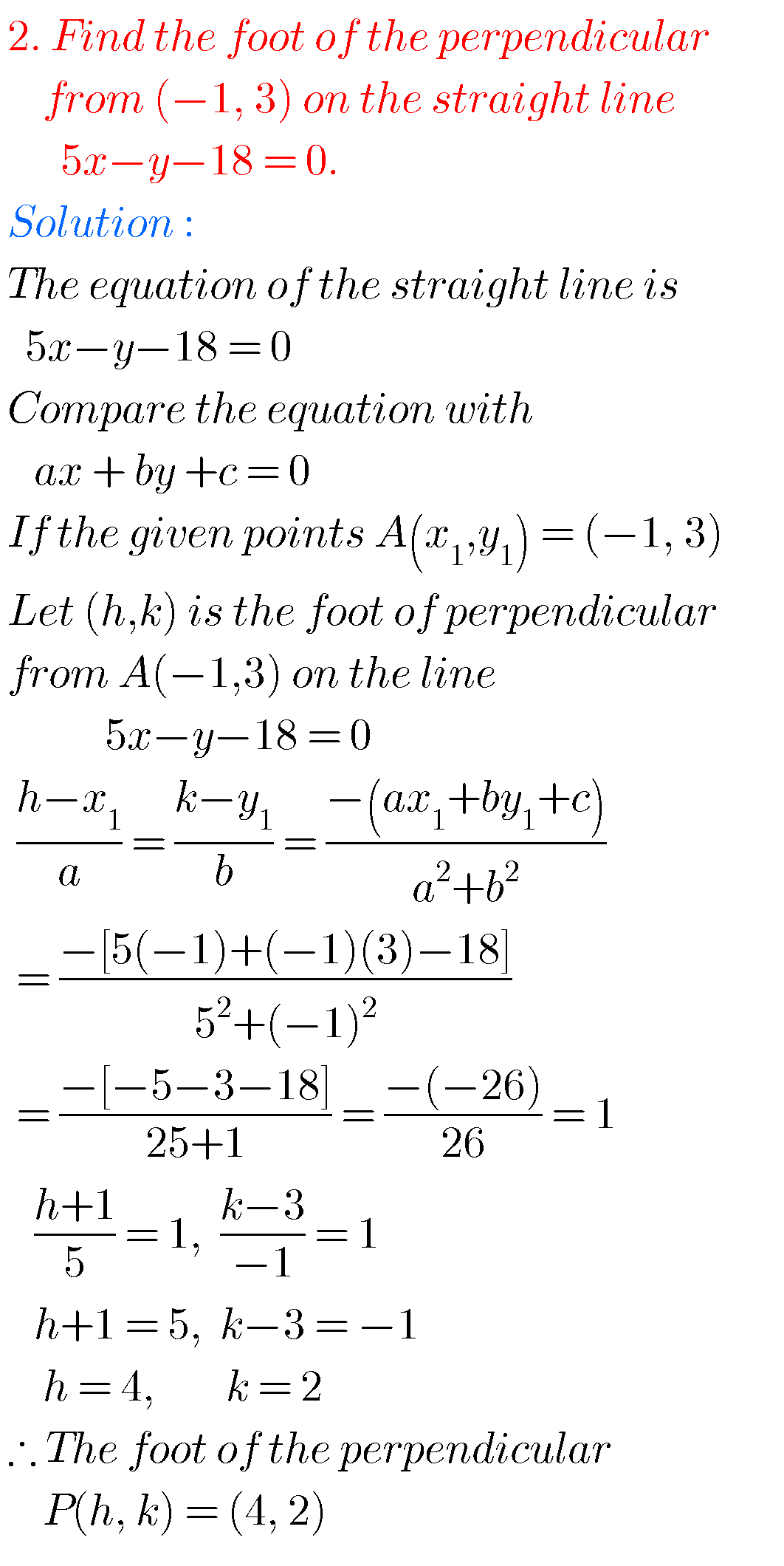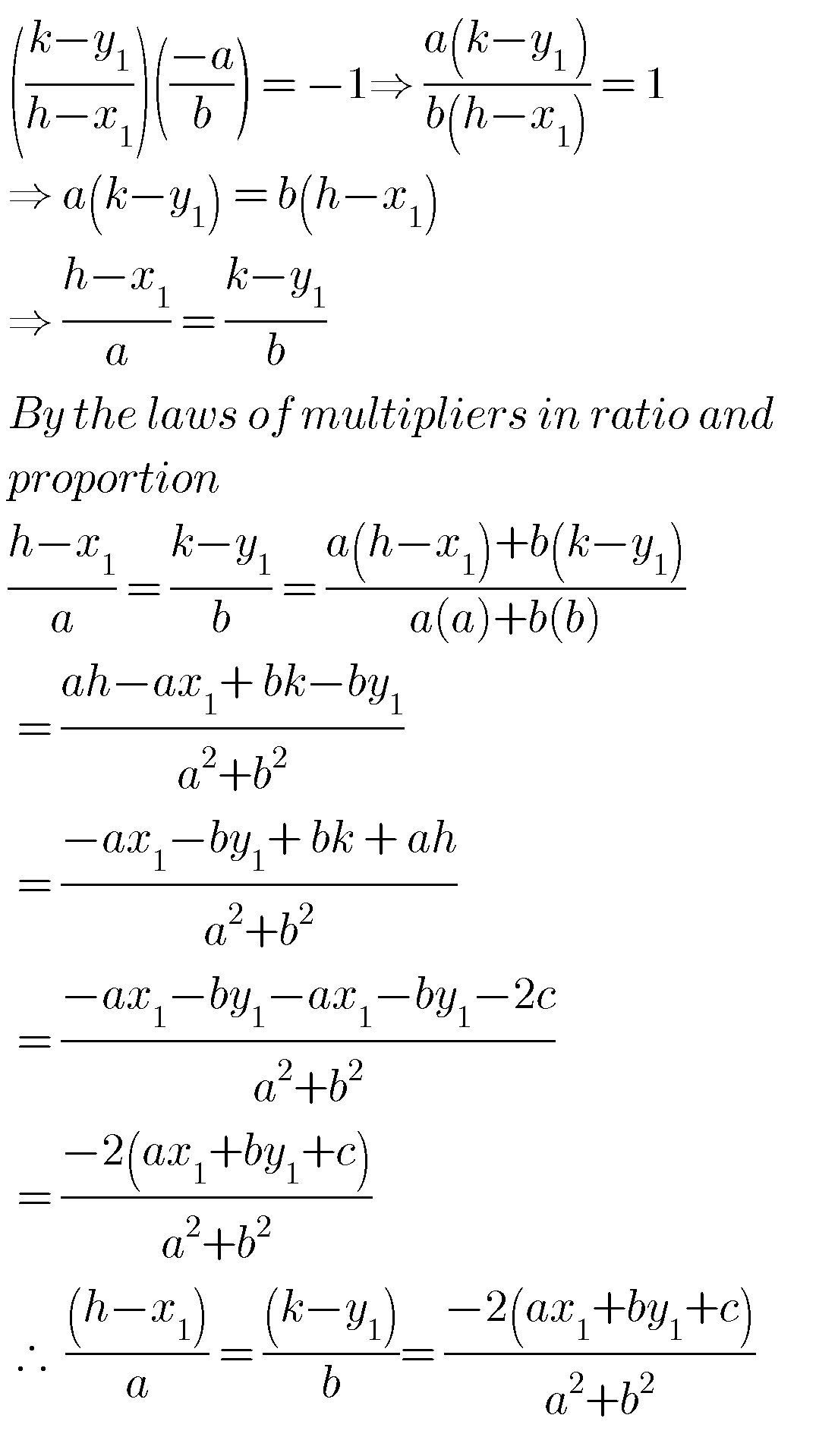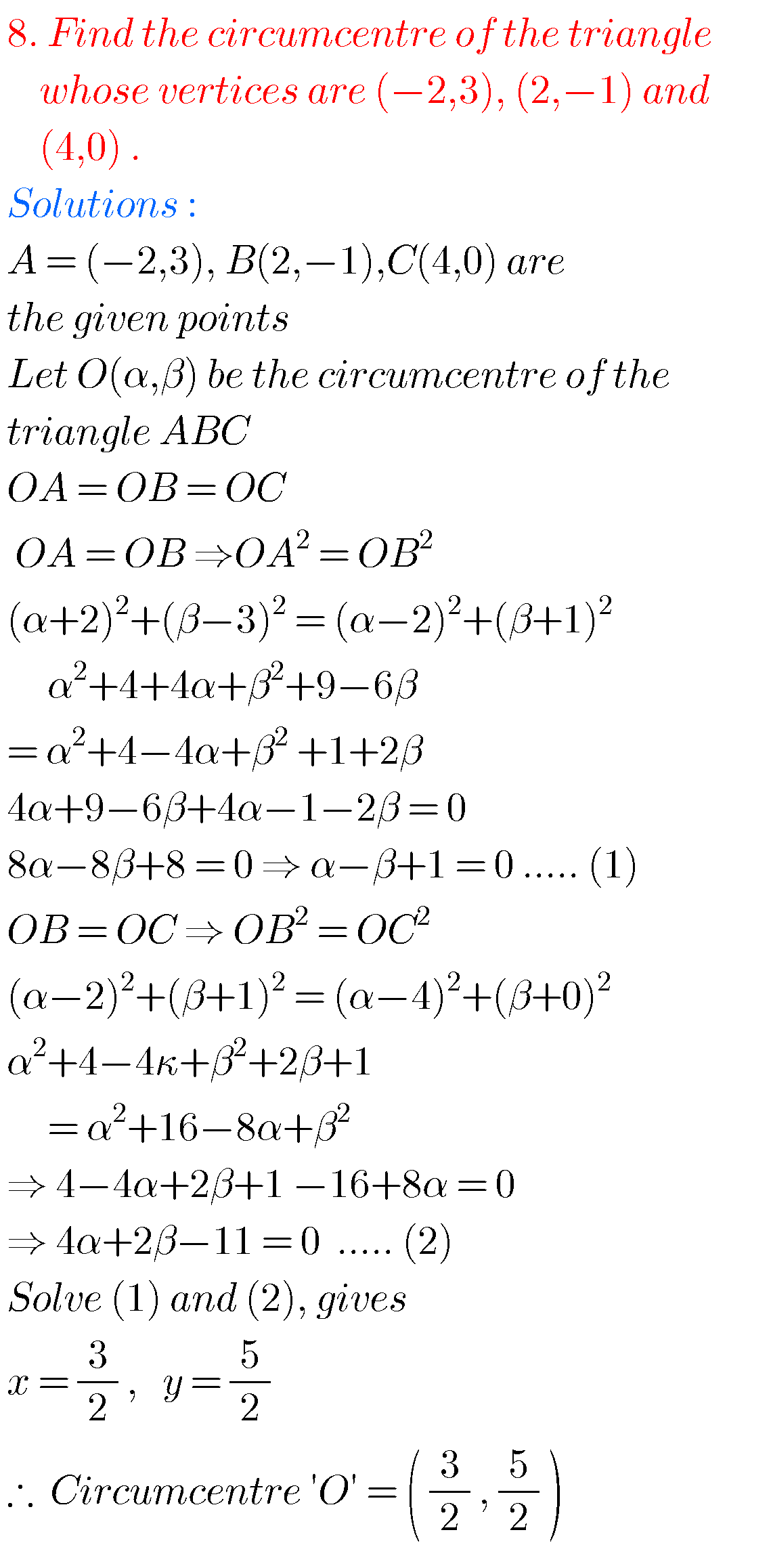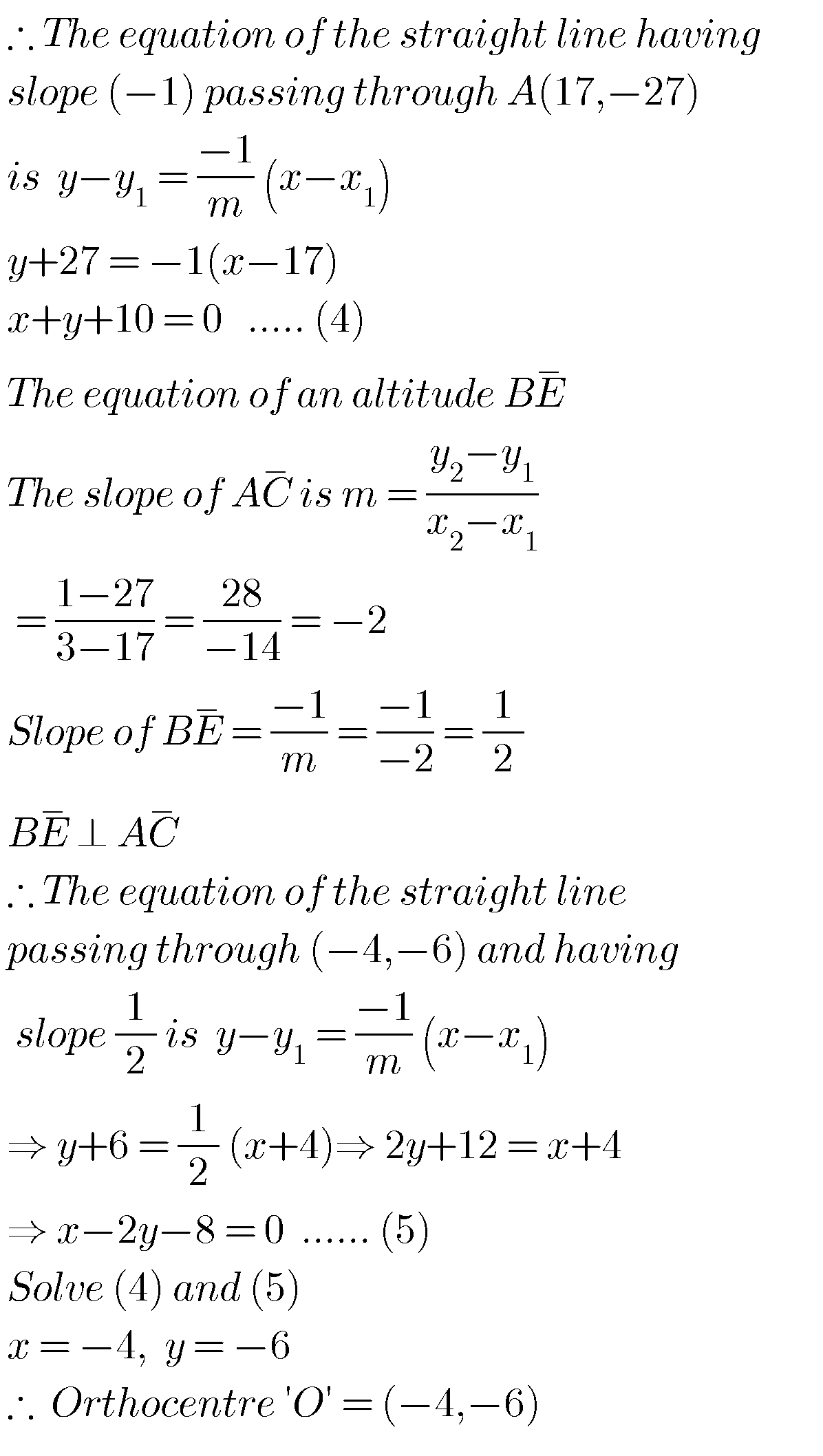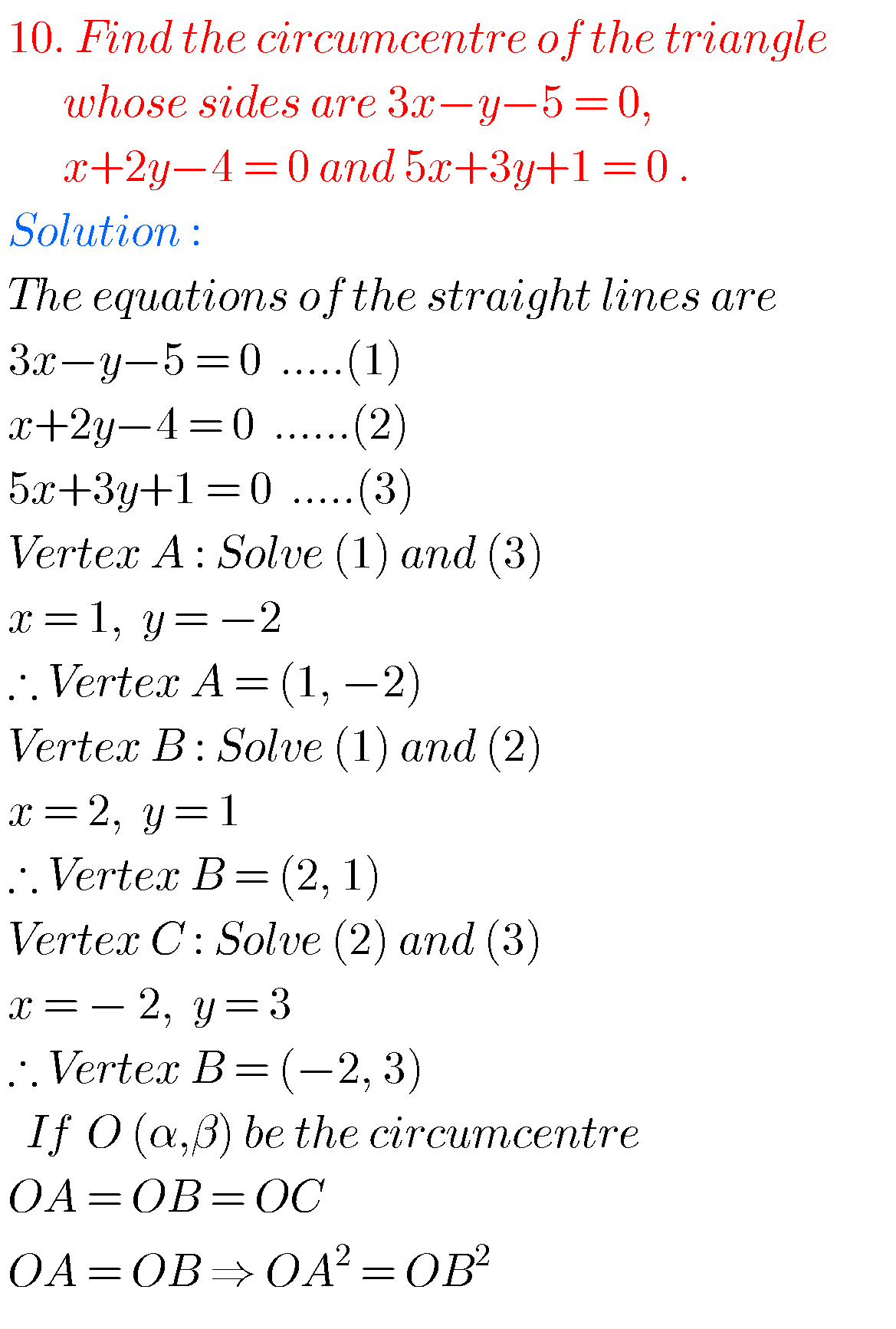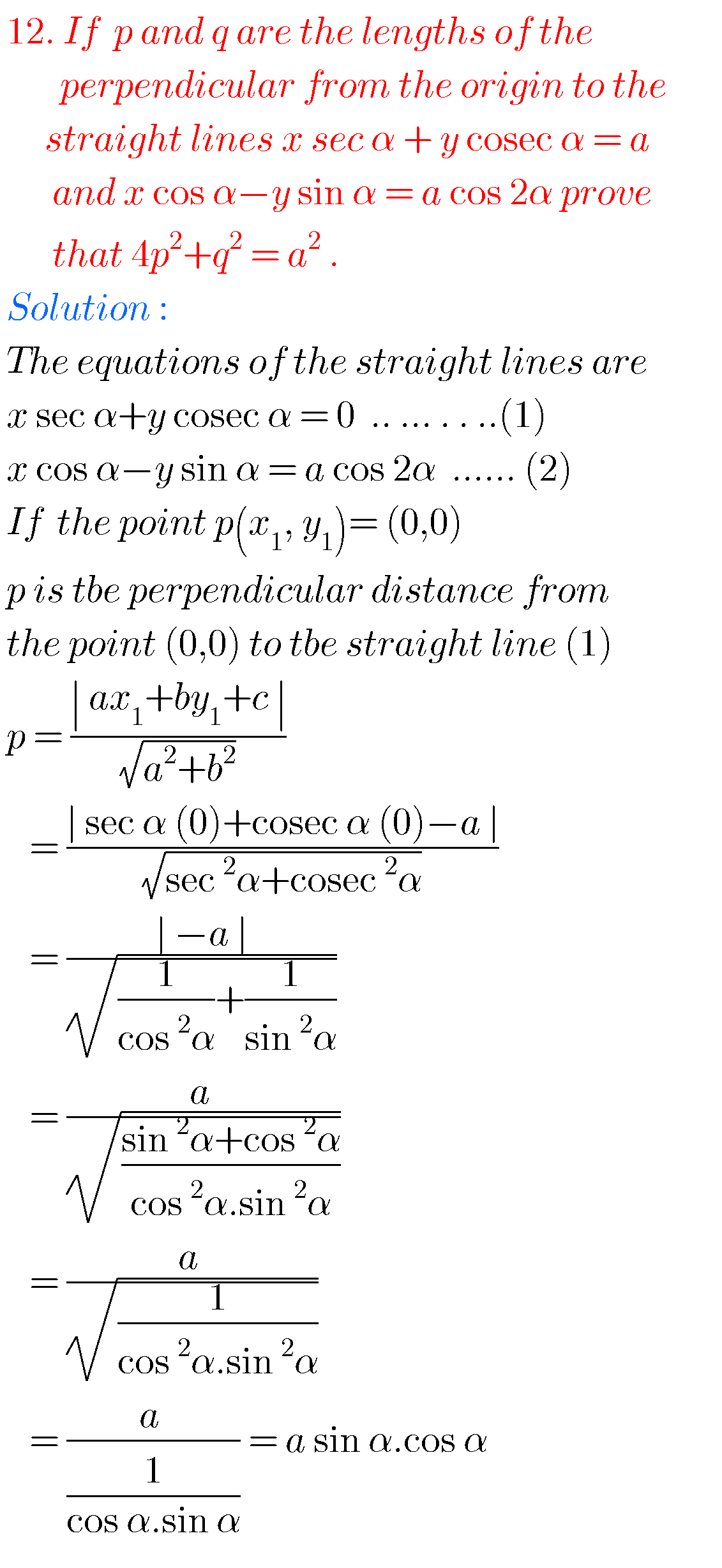Note : Observe the solutions and try them in your own methods.

Maths real numbers solutions class 10

Intermediate maths 2a textbook solutions

You can see solutions for Inter Maths IIB

1. Circle

3. Parabola

4. Ellipse

You can also see solutions for Inter Maths IIA

For examination purpose you can see

Complex numbers

De Moivre’ s Theorem

### One Comment

1.Sarayu September 18, 2019 at 7:49 pm - Reply